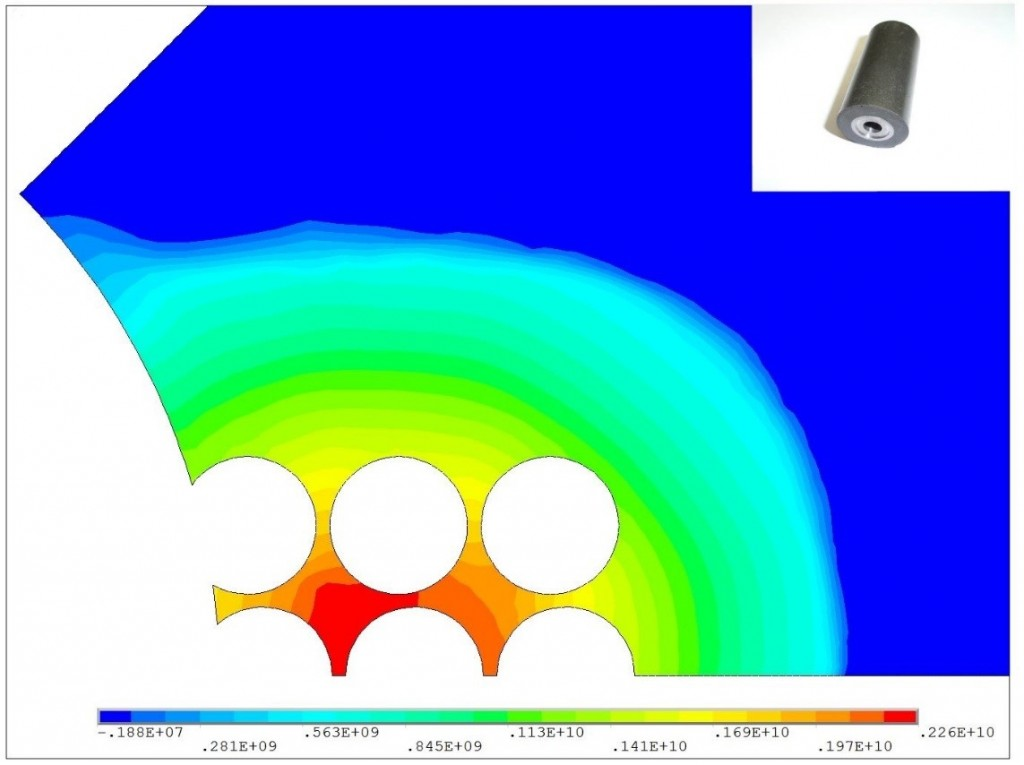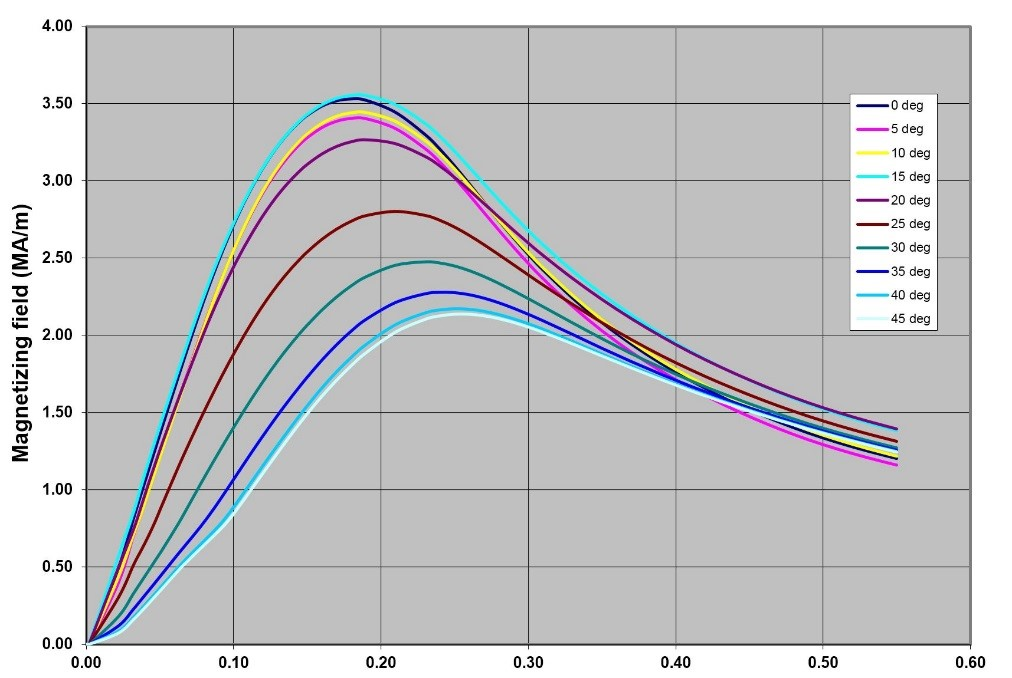# How It Feels To Detect Simulation Errors

By Thomas Schliesch, Head of Research & Development | Max Baermann GmbH

Recently I took an old simulation of a magnetizing coil to apply it with a few modifications to a new magnet. This kind of simulation used a coupling between the coil itself and the feeding condenser discharge circuit, which was controlled by fairly long scripts for the FEM software. I had demonstrated that special coil together with the calculation of a related isotropic magnet as one subject of my presentation at the Magnetics 2012 conference . Some of the results were also shown again at the Magnetics 2016 in another context. When flipping through the mentioned scripts to make the changes for the new magnet, suddenly something caught my eyes which made me get confused first, then later made me mad. Oh my! I found an error in one script! And I had presented this stuff at the Magnetics conference! I started to feel really distressed and ashamed. Did I really present wrong results? Was the whole subject probably basically wrong by this mistake or was it a minor flaw only? I immediately took all the related files and calculations, nervously checking if there was really a mistake and after proving it, I had to admit: Yes there was. At Once I started to recalculate all the different versions and aspects which I had presented at the 2012 meeting.

Let us get a bit more into detail. Figure 1 shows the core of the magnetizing coil, which was used for a four pole cylinder magnet. There are four bundles made of nine wires each, but shown is only 1/8 of the whole assembly here. The core was made of bulk steel material leading to eddy currents during the magnetizing pulse. Figure 1 illustrates the eddy current density at the raising branch of the discharge current (already in the revised version). What I showed in 2012 at the conference was the simulation of the coil/circuit system and the calculation of the resulting polarization distribution by application of a useful hysteresis model. Additionally, I presented different stages of model refinement there. The simplest assumption was, that polarization is determined by the magnetizing fields at the time of the maximum of the electrical current. Another version calculated an average time of field maxima inside the magnet and took this as the relevant time to build up the magnetization distribution. A more refined access was to use the local field maxima inside the material. Using these local field maxima and in addition taking into account rotations of magnetization during the field decay, was the final and most refined version.Figure 1. Eddy currents at t=0.08ms in a magnetizing coil for a four pole cylinder magnet (corrected version).

The above mentioned mistake happened at the coil simulation, when giving in symmetry factors 2, taking into account the modelling of the three half conductors at the bottom of the 1/8 assembly of Figure 1. Instead of using it for the bottom wires I inadvertently placed them at the top wires. This led to a completely non uniform distribution of current inside the wires, i.e. the three wires at the central row had a current which was much too large, whereas the others had a current much too low. I think it is comprehensible that by finding this mistake I was in doubt about the adequacy of all my results of the related investigations. Now let us come to the results of the revised version, which I now used with the symmetry factors at appropriate locations.

For the eddy current densities at time 0.08 ms, which I had shown in 2012 and as being depicted in Figure 1, only minor differences could be observed. This is valid both in regard to the colors as well as in respect of the figures at the bottom legend. But differences could be observed fairly well at the local time distribution of the H-fields inside the magnet. Fig. 2 shows the vector sum of H of the erroneous calculation as a function of time for different angular positions. The radial position is 0.2 mm inside the magnet. The zero angle correlates to the bottom of Figure 1, i.e. it is located in front of the central row of wires. Figure 3 depicts the same results for the corrected simulation. Figure 2 shows a monotone decrease of field maxima with increasing angular position. This is originated by the fact, that in the incorrect coil calculation the central conductors have a much larger electrical current compared to the other ones. Instead of that in Figure 3 the field maxima are at a comparable level within the first 20°, which depicts reality in an adequate way.Figure 2. Magnetizing field as a function of time 0.2 mm inside the magnet, erroneous simulation with wrong distribution of total current in conductors.

The aim of the coil-magnet analysis I had presented at the Magnetics show was to check if the behavior of an isotropic, pulse magnetized magnet could be predicted well by use of relatively simple simulations of the magnetizing process. Now after finding the mentioned mistake the exciting question came up: How is the impact of the correct coil simulation in comparison to the erroneous one on the behavior of the magnet? Were the relatively optimistic results from 2012 wrong and is the simulation of pulse magnetized, isotropic magnets not that simple as I assumed? To check the mentioned approaches to simulate polarization built up, in 2012 the radial field component of the magnet was calculated and compared to measured values as an indicator of simulation quality.  Figure 4 shows respective results in front of one pole for the old simulations. The blue curve depicts measured Hall probe values at a radial surface distance of 0.35 mm. Green is when magnetization is calculated at the current maximum of the feeding circuit, orange when a time average of local field maxima is used. Yellow is for the case when local field maxima are applied and red when in addition rotations of magnetization are taken into account by a sort of Stoner-Wohlfahrt model of hysteresis.Figure 3. Magnetizing field as a function of time 0.2 mm inside the magnet, revised simulation.Figure 4. Results of different simulation models of the magnetizing process, based on erroneous coil simulation from 2012. Radial flux density at a distance of 0.35 mm from magnets curved surface area.

Figure 5 depicts respective results when using the adequate coil calculation. When looking at these results the first time, it took a load off my mind! Results are qualitatively the same and simulated values are even closer to measurements than before. Additionally to the pictures shown here I had presented figures about the distribution of polarization rotations in 2012. These figures also kept to be nearly the same with the new coil analysis.About the Author